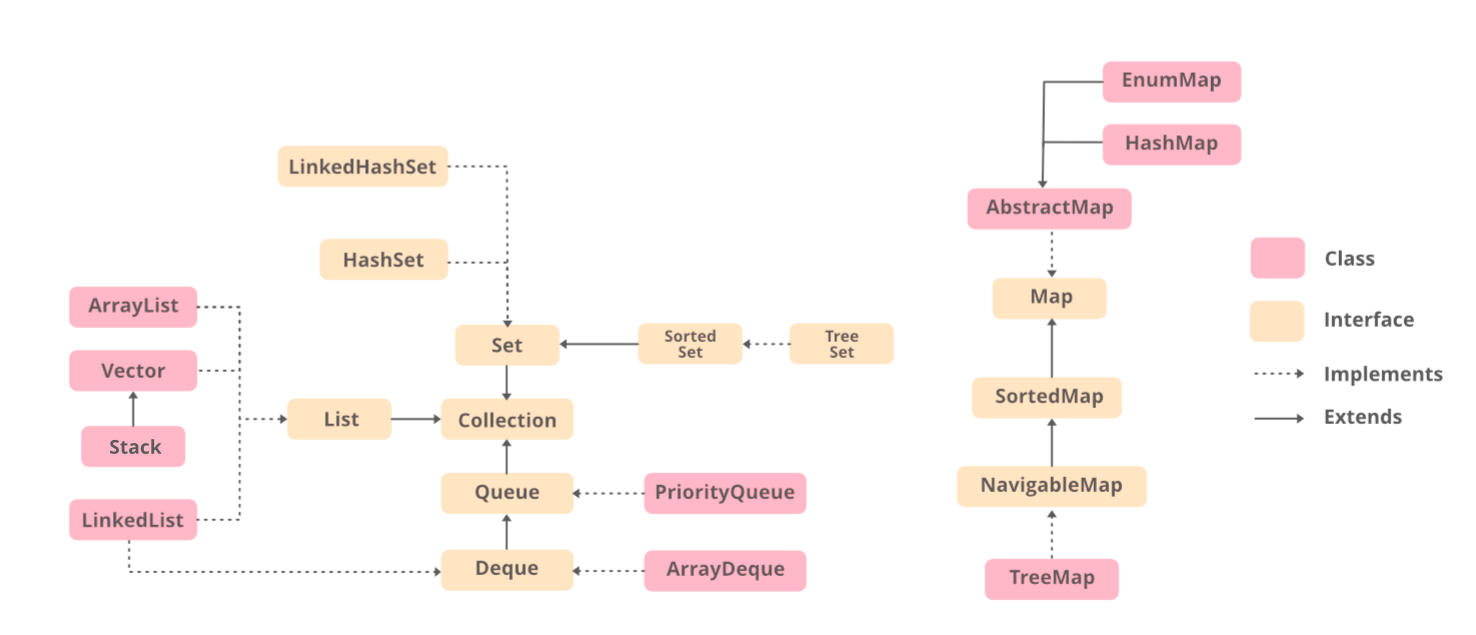Open In App

# Difference between List and ArrayList in Java

A Collection is a group of individual objects represented as a single unit. Java provides Collection Framework which defines several classes and interfaces to represent a group of objects as a single unit This framework consists of the List Interface as well as the ArrayList class. In this article, the difference between the List and ArrayList is discussed.List is a child interface of Collection. It is an ordered collection of objects in which duplicate values can be stored. Since List preserves the insertion order, it allows positional access and insertion of elements. List interface is implemented by the classes of ArrayList, LinkedList, Vector, and Stack. List is an interface, and the instances of List can be created by implementing various classes.

Geek if the above said sounds confusing then refer to the image below where you can easily spot the hierarchy and the List interface consisting of classes prior to landing upon implementation of List interface.Example

## Java

 `// Java program to demonstrate the``// working of a List with ArrayList``// class` `// Importing all utility classes``import` `java.util.*;` `// Main class``public` `class` `GFG {``  ` `    ``// Main driver method``    ``public` `static` `void` `main(String[] args)``    ``{``        ``// Creating an object of List class``          ``// Declaring an object of String type with``        ``// reference to ArrayList class``        ``// Type safe list``        ``List al = ``new` `ArrayList();``      ` `        ``// Adding elements using add() method``        ``// Custom input elements``        ``al.add(``"Geeks"``);``        ``al.add(``"for"``);``        ``al.add(``"Geeks"``);` `        ``// Print and display the elements in``        ``// ArrayList class object``        ``System.out.println(al);``    ``}``}`

Output

`[Geeks, for, Geeks]`

Now dwelling on the next concept of ArrayList in java. So ArrayList is basically a part of the collection framework and is present in java.util package. It provides us with dynamic arrays in Java. This class implements the List interface. Similar to a List, the size of the ArrayList is increased automatically if the collection grows or shrinks if the objects are removed from the collection. Java ArrayList allows us to randomly access the list. ArrayList can not be used for primitive types, like int, char, etc. We need a wrapper class for such cases. The following is an example to demonstrate the implementation of an ArrayList.

Syntax:

`new ArrayList();`

This simply creates a new memory in the heap memory. In order to access the object, we need a reference variable as it is a thumb rule in object-oriented programming.

`ArrayList obj = new ArrayList();`

By far we have only created an object but it is not defined what type of elements will be there in our ArrayList object. So as usual we will be using identifiers to pass String type, Integer type, both, or some other types. It is as shown below.

```ArrayList<Integer> obj = new ArrayList<>();
ArrayList<String> obj = new ArrayList<>();```

Note: While adding the elements to ArrayList if we do add element at an index say ‘i’th then in our ArrayList all the elements shifts towards right where the previous element which was at ‘i’ th before addition will now be at ‘i+1’ th index. It will not be replaces just unlikely as we do see in arrays.

So by now, we are done with the understanding of declaring and how to initialize a List via an above understanding of syntax, let us now implement the same within a program to get a better understanding.

Example:

## Java

 `// Java Program to Demonstrate``// Working of an ArrayList class` `// Importing all classes from java.util package``import` `java.util.*;` `// Main class``class` `GFG {` `    ``// Main driver method``    ``public` `static` `void` `main(String[] args)``    ``{``        ``// Creating an ArrayList of String type``        ``// Type safe ArrayList``        ``ArrayList al = ``new` `ArrayList();` `        ``// Adding elements to above object created``        ``// Custom input elements``        ``al.add(``"Geeks"``);``        ``al.add(``"for"``);``        ``al.add(``"Geeks"``);` `        ``// Print and display the elements of ArrayList``        ``System.out.println(al);` `        ``// adding element at index where``        ``// element is already present``        ``al.add(``1``, ``"Hi"``);` `        ``// Print and display the elements of ArrayList``        ``System.out.println(al);``    ``}``}`

Output

```[Geeks, for, Geeks]
[Geeks, Hi, for, Geeks]```

Now let us discuss the differences between two classes in java as discussed above are List vs ArrayList classes, they are shown below in the tabular format below as follows for clear thin-line understanding.